# Basic Matrix OperationsHaving difficulties with matrix problems while doing math homework? In such situation it’s a great idea to ask your math tutor for help because any misunderstanding at the start can result in greater problems at your midterm and final.  Yet another great idea is to go through tutorials on the topic to straighten your knowledge on the matter. We prepared the series of articles and video tutorials covering basic concepts, also we considered several examples on matrices, similar to those in your homework, more serious assignments and exam tasks. We’ll explain how matrices are used in general, which operations are allowed to perform with matrices and how to calculate the determinants. Let’s get started.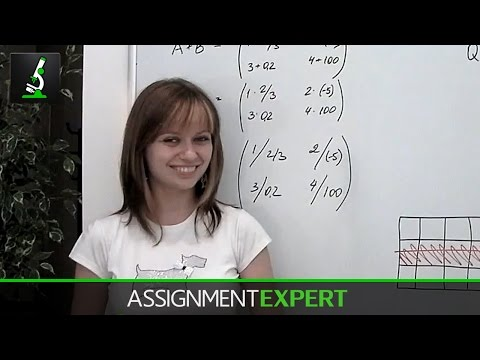In this article we’re going to give you the idea of how to deal with matrices and perform some basic operations with them.

First of all, what is matrix?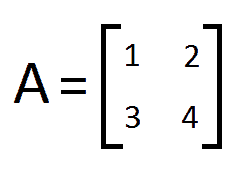Basically, matrix is just a table with numbers in its cells. These numbers are called elements or entries of a matrix. So if we put the numbers in table form and then enclose them into brackets… whoaaaa, we have a matrix. Just as matrices A and B: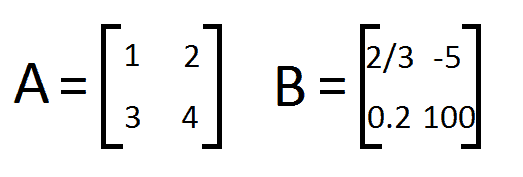Each number in the matrix is called an entry or an element of the matrix. For example, numbers 1,2,3,4 are elements of the matrix A. Matrix has rows and columns, rows go from left to right, columns go up-down.

Obviously, matrices can be of different size depending on the number of rows and columns they have. For example, matrices A and B are of size 2X2 where the first number stands for number of rows and the second – for number of columns. Consider matrix P: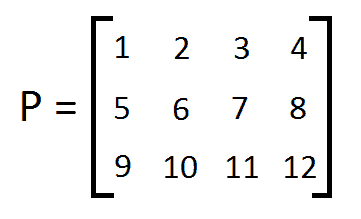It has 3 rows and 4 columns so this matrix is of size 3X4. In homework tasks you can face differently sized matrices, say 3X5, 3X3 and so on.

If matrices are of the same size, we can easily define their sum or difference simply by adding or subtracting the corresponding entries. As we can see, A and B are of the same size, they both contain two rows and two columns. Therefore we can easily find their sum: we take the numbers lying in the same positions and add them.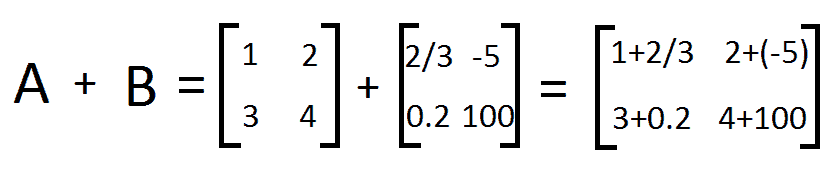The entries of the resulting matrix are the sums of corresponding entries of addend-matrices.

## Matrix Subtraction

In similar way we can perform the subtraction of matrices by subtracting corresponding elements of the second matrix from corresponding elements of the firs: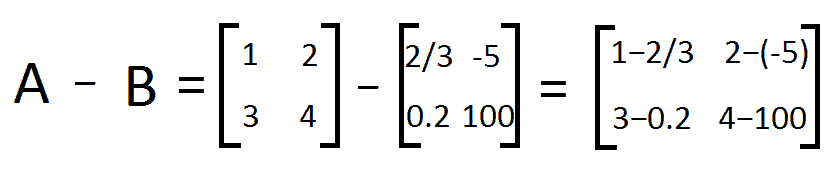If you need to add or subtract two matrices in your homework, don’t forget to check if they’re of the same size! Only in such case the corresponding operation can be done.

## Multiplication by a number

To multiply matrix by a number we simply multiply each entry of the matrix by this number. For example, let’s multiply our matrix A by the number 7: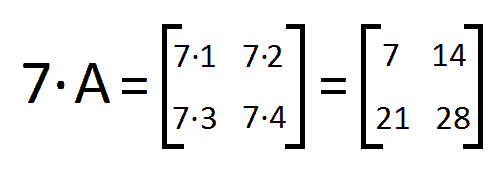Thus, each entry of the resulting matrix equals to the corresponding entry of matrix A multiplied by 7.

## Matrix Multiplication

Apparently, following the same logic, we could introduce multiplication and division of matrices, so according to our algorithm we would take numbers staying in the same position and find their product or quotient. But these expressions are not exactly correct. Although such element-by-element multiplication and division do take place, the matrices resulting from such procedures should never be called a product or a quotient. You may ask but how can those be obtained?  We do have an answer for that and we’ll reveal it in a little while.

Now we move to the most interesting part of this article – doing the multiplication of matrices which results into the product matrix.

First of all, you always need to remember that multiplication of matrices can only be done on one condition: the number of columns of the first matrix being multiplied must equal to the number of rows of the second matrix being multiplied. Just keep this in mind, soon we’ll provide an explanation.

So if we have the matrix A of the size nXm, which means it has m rows and n columns, it can only be multiplied by a matrix containing m rows. So matrix B of size mXl is the perfect choice for this case, because it has m rows and l columns.

Multiplying these matrices, you will always get a resulting matrix AB of size nXl.

For better understanding of the whole idea of matrix multiplication let’s consider the following picture: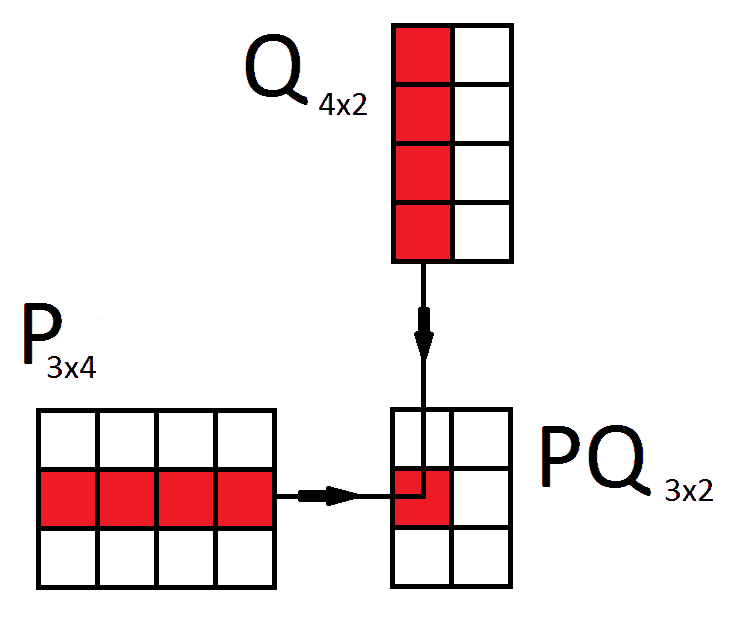Here we have two matrices: the first matrix P of the size 3X4 and the second Q of the size 4X2. The product matrix PQ of these two will be of the size 3X2.

Each entry of the product matrix lying on the crossing of the certain row of the first matrix and certain column of the second matrix equals to the sum of the products of the corresponding elements of these row and column. It does sound a little messy and twisted. So once again, in order to calculate the highlighted element of the product matrix PQ first you need to find the appropriate row in the first matrix being multiplied. And then the appropriate column in the second matrix being multiplied. And then you start multiplying elements of these lines component-wise. So you multiply the first element of the row by the first element of the column, then the second element of the row by the second element of the column and so on. Finally sum up the products.

This is exactly the reason why the number of columns of the first matrix must match the number of rows of the second matrix, otherwise not all elements would have corresponding pairs and therefore procedure of finding product would fail.

Now let’s multiply two matrices.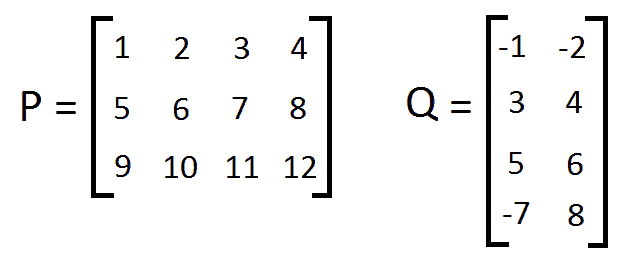Here’s matrix P of size 3X4 and matrix Q of size 4X2? As we see matrix P has 3 rows and 4 columns, and Q has 4 rows and 2 columns. Perfect match for multiplication!

So we’re finding the appropriate row – the first row of matrix P, and the appropriate column – the first column of matrix Q and multiply them component-wise.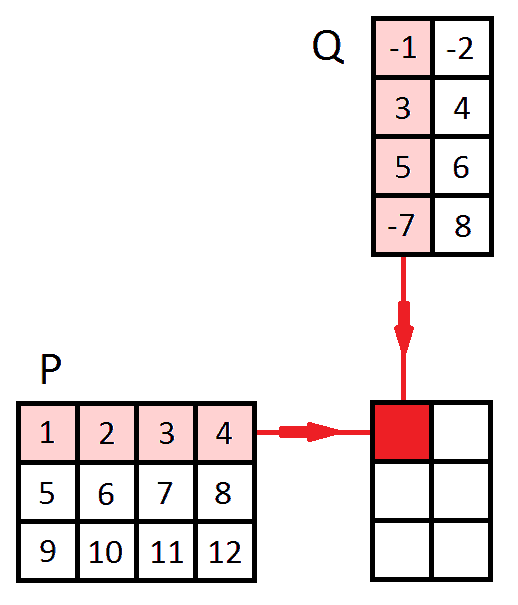The element standing in the position 11 (in the first row and in the first column) in the resulting matrix:

$$1\cdot(-1)+2\cdot3+3\cdot5+4\cdot(-7)=8$$

To calculate the element 12 we use the same row of the first matrix P, but the second column of the matrix Q:

$$1\cdot(-2)+2\cdot4+3\cdot6+4\cdot8=56$$

Now we calculate the elements of the second row of product matrix. We’re now going to use the second row of the first matrix P.

Element 21:

$$5\cdot(-1)+6\cdot3+7\cdot5+8\cdot(-7)=-8$$

To find element 22 we use the same row of P, but now the second column of the matrix Q:

$$5\cdot(-2)+6\cdot4+3\cdot7+8\cdot8=120$$

And finally, the third row of the resulting matrix. For that we need to use the third row of matrix P.

Element 31: the third row of P multiplied by the first column of Q

$$9\cdot(-1)+10\cdot3+11\cdot5+12\cdot(-7)=-6$$

And at last the element 32 of the resulting matrix: we use the third row of P and the second column of Q

$$9\cdot(-1)+10\cdot3+11\cdot5+12\cdot(-7)=184$$

Ok, now we can fill in the entries of the resulting matrix PQ we’ve just calculated: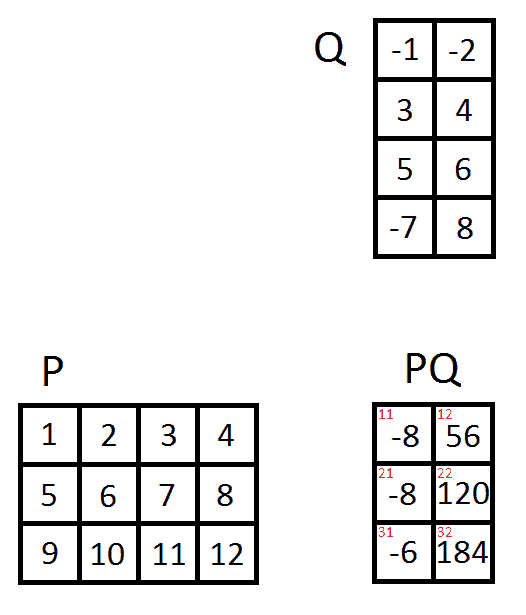So, matrix P of the size 3X4 multiplied by matrix Q of the size 4X2 results in matrix PQ of the size 3X2: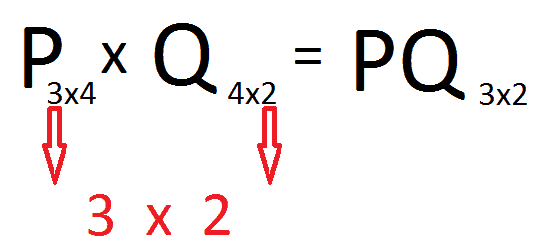Now you might ask why haven’t we said anything about division of matrices? Well, actually it’s performed this way:

$$A / B=A\times \frac{1}{B}=A\times B^{-1}$$

where $$B^{-1}$$ is an inverse matrix. In fact we don’t divide matrices, instead we multiply by inverse matrix. That’s not a short story to tell, so about inverse matrices in the next article.

0 Shares
Filed under Math.